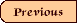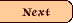Part 1
Mathematics, Logic & Computers

1.1  Mechanical computers
1.2  Abstract mathematics
1.3  Boolean logic
1.4  Reducing mathematical relationships to abstract Boolean logic
1.5  Physical implementation of Boolean logic
1.6  Universal computation

Part 2
Cellular Automata Computers

2.1  Basic structure and operation of a CA
2.2  Useful things to do with a CA

Part 3
Banks' Proof of Universality in 2-dimensional, 5-neighborhood CA

3.1  Rules of change
3.2  The wire and signal
3.4  The fan out junction
3.5  The clock
3.6  The logic element
3.7  The NOT gate
3.8  The NOR gate

Part 4
Epilog

4.1  Manipulation of information by whatever means
4.2  The machine within the machine
4.3  The dependent automaton
4.4  Progress in understanding computers and cellular automata
Acknowledgements

Back to abstract

Go to BottomLayer home

Commentary on Edwin Roger Banks, "Information Processing and Transmission in Cellular Automata," Banks' Ph.D. thesis, 1971 (MIT, Dep't of Mechanical Engineering).  By Ross Rhodes.

## Part 1  Mathematics, Logic, and Computers

In his doctoral thesis, Banks takes a great deal for granted.  After all, he is writing for computer scientists and engineers.  This commentary is intended for a more general audience.  Accordingly, we will begin with a review of some of the fundamental aspects of mathematics, logic, and computers.  If you already know this stuff, please skip ahead to Part 2 which discusses cellular automata.  If that is also familiar to you, you may jump straight to Banks' starting point at Part 3.

### 1.1 Mechanical Computers

In modern parlance, a computer is a machine that computes. In earlier eras, a "computer" might have been a research assistant who was given a math problem and asked to deliver the solution by the next day's class. The machine nature of modern computing is important to an understanding of its role in mathematics, and ultimately to an understanding of Roger Banks' proof.

The concept of mechanical computing - as opposed to the research assistant's deep thoughts - may be traced to Alan Turing's analysis of mathematics itself, developed in 1936. Turing took up the challenge of trying to determine whether a mathematician could predict in advance the outcome of some mathematical problem. This challenge required that he find a simple way to describe the underlying nature of mathematics itself, such that his description would apply to any and all and every mathematical problem whatsoever.

### 1.2 Abstract Mathematics

Whereas the engineer might state the empirical fact that a particular brick weighs two pounds and a particular rock weighs four pounds, the mathematician will assert the abstract relationship among these two items: the rock is twice as heavy as the brick. If this turns out to be a fundamental quality of bricks and rocks, the mathematician will inform the engineer that the weight of one rock equals the weight of two bricks. In caveman speak, this may be expressed as "Rock equals two brick," with the understanding that we are talking about weight.

To further simplify matters, and to avoid the need to translate for visiting cavepersons, the mathematician will express the relationship symbolically: R = 2B.

Now we have something magical for the mathematician to play with, because if "rock equals two brick" then it is also true that "brick equals one-half rock." B = R/2. Eureka! What is more, if we divide Rock by Brick, we will always come up with the number 2; that is, "rock divided by brick equals 2," or R/B = 2. Wow! A physical constant!!

At this point, the engineer objects. "What do you mean divide rocks by bricks? How can you do that? What are you talking about?" But the mathematician is heedless, because he is not really dealing with rocks and bricks any longer, he is dealing with abstract relationships among Rs and Bs.

### 1.3 Boolean Logic

The rules for accomplishing these operations were well established by Turing's day. In 1854, George Boole had investigated "the laws of thought" - literally analyzing how people think and rationalize and come to logical conclusions, in particular with respect to the symbolic logic of mathematics. In logic, every statement is either true or false, which means that the logician has to deal with only these two possibilities. Yes or no; true or false.

This principle holds even in a world of many variations. For example, an object may be a rock, a brick, a cement block, or some other thing. We may still conduct our inquiry by asking a series of yes-or-no questions: Is it a rock? Yes or no. Is it a brick? Yes or no. Is it a cement block? Yes or no. Another way to accomplish the same thing is to state the conclusion (tentatively) and then determine whether the conclusion is true or false. It is a rock - true or false? It is a brick - true or false?

Boole's simple description of the whole of human thought turned out to be just the kind of thing that mathematicians love best, because it allowed the thinker to get away from rocks and bricks to focus on the relationships among statements considered in the abstract.

### 1.4 Reducing Mathematical Relationships to Mechanical Boolean Logic

As Turing contemplated these mathematical and logical processes, he saw his task as reducing all of mathematics to a series of step-by-step operations that involved nothing more than comparing two yes-or-no, true-or-false items and then moving on to the next step according to the relationships implied by the math. If it is really that simple, then we should be able to do away with the thinker altogether and instead devise a machine to carry out all of the comparisons. The thinker could then sit back and let the machine do all of the work implied by the complicated mathematical formula - because the thinker is only interested in the final result, which is whether the whole mathematical formula is ultimately true or ultimately false. Turing imagined that the thinker could kick his feet up until the machine finished its work, at which time a bell would ring and the machine would have the simple yes/no answer at the end of all of its (perhaps billions of) simple yes/no comparisons. [note 1]

The particular type of machine envisioned by Turing has not proven to be practical as a general purpose computer. However, Turing had laid the foundation stone by demonstrating that a machine could carry out all of the steps necessary to solve any mathematical problem that could be solved.

Very soon after Turing described his concept of reducing all of human thought to simple operations that could be carried out by a machine, the engineers of this world realized that there are actually ways to accomplish this. That is, we can build a device that will compare two yes-or-no statements (input) and spit out another yes-or-no statement that depends on the result of the comparison (output). The design of these general purpose universal computers was fairly simple, and it was quickly shown that they could emulate Turing's own design, that is, they could be configured to accomplish anything that Turing's imaginary machine could accomplish. By the elegance of logic, it was enough proof to show that the new machines were equivalent to Turing's machine. At that point, all of Turing's further proof that his machine was universal could be appropriated to the new machines, thereby proving that they, too, were universal.

### 1.5 Physical Implementation of Boolean Logic

In fact, there are many ways to build the machine. All we need is a gate (and a gatekeeper).

The inputs will approach the gate like sheep and goats. The gatekeeper will determine whether the two animals coming to the gate are two sheep; a sheep and a goat; or two goats. And the gatekeeper will send either a sheep or a goat along to the next gate (and gatekeeper), depending on what combination of animals originally approached his gate.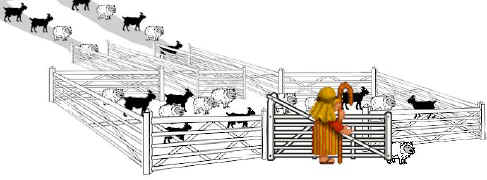Fig. 1.1  Baah-lean logic.

(If it were not for the fact that we want to eliminate humans from the process, we could actually build a perfectly serviceable computing machine entirely out of pastures, paths, sheep, goats, shepherds, and wooden railings.)

Perhaps the most common mechanical implementation at the moment is to use electricity running down wires. The two inputs are simply two wires going in to the "gate." The wire of an input will be "true" if it is conducting electricity (i.e., the circuit is closed and therefore turned on), and "false" if it is not conducting electricity (i.e., the circuit is open and broken and therefore off). At the "gate," these two wires are routed through electrical devices such that a signal will be produced by the entire set up (as output) only if the specified conditions exist at the input wires.

For example, suppose we want to make an electrical circuit that will only give the answer/output "true" if both inputs are "true." This will function as an AND logic gate, i.e., for two inputs A and B, the circuit will be active if and only if A is true AND B is true. One way to accomplish this is as follows:

1.  Devise a switching mechanism, such that when the circuit is inactive/off/false the switch will be open; and when the circuit is active/on/true the switch will be closed.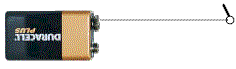Fig. 1.2  Battery-operated switch in the off position.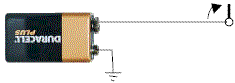Fig. 1.3  Battery-operated switch in the on position.
2.  Place the switches along the wire of a master circuit.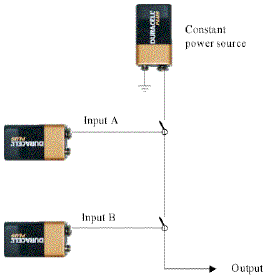Fig. 1.4  Battery-operated AND gate. If the inputs are "on" they will close their switch. Both inputs must be "on" (both switches must be closed) to complete the constant power circuit. The output will only be "on" (true) if both A and B are "on" (true).

That is all we need to do to compare two inputs and decide mechanically whether they are both active/on/true. With this circuit design, the master circuit will be broken whenever either of the inputs is inactive/off/false, because either switch will break the master circuit if it is open. Only if both switches are closed will the master circuit be completed, which will allow an active/on/true signal to emerge.

There are at most seven logic gates necessary to a complete mechanical system implementing Boolean logic, which is to say implementing the entire laws of human thought. These are:

1.  The NOT gate. This is the only single-input gate, and it simply reverses the input.  For this reason, it is also called an inverter. Output is true if the input is false, and output is false if the input is true. Very contrary.

It is quite simple to devise a gate that will accomplish this inversion. For example, we can readily imagine reversing the switching mechanism described above, simply by moving the wire slightly, like this: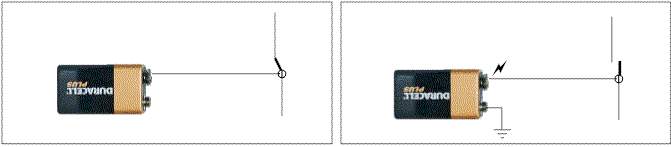Fig. 1.5  Circuit is closed (on) when input is off; circuit is open (off) when input is on.

We can put this reversing switch in the master circuit, so that whenever the input is "on" the master circuit will be broken (off), and vice versa.  We can thus reliably invert the input from on to off, and from off to on.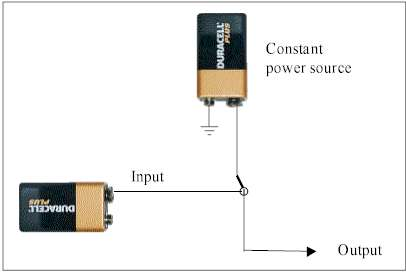Fig. 1.6  Battery-operated NOT gate to invert the input so that the output is its opposite.

2.  The AND gate. This is a two-input gate. Output is true if (and only if) both inputs are true, as illustrated earlier.
3.  The OR gate. This is a two-input gate. Output is true if either (or both) of the inputs is true.

Here is a simple implementation of the OR gate, using our basic (non-inverting) switch: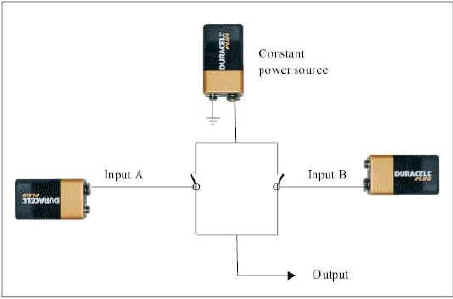Fig. 1.7  Battery-operated OR gate.  Output is true if either A or B is true.
Because we have the NOT gate, we don't really need to build the following gates which can be constructed by various combinations of the first three gates. Nevertheless, these gates are necessary to the complete system of Boolean logic:

4.  The NAND (i.e., not AND) gate. This is a two-input gate. Output is false if (and only if) both inputs are true. You can see that this is simply an AND gate coupled with a NOT gate which inverts the output/answer.

5.  The NOR (i.e., not OR) gate. This is a two-input gate. Output is false if either (or both) of the inputs is true. You can see that this is simply an OR gate coupled with a NOT gate which inverts the output/answer. [note 2]

Finally, there are two more logical operations which, again, can be constructed by using a series of the foregoing gates, but which are also recognized as necessary to a complete implementation of Boole's system of logic:

6.  The XOR ("eXclusive" OR) gate. This is a two-input gate. Output is true if one of the inputs is true and the other is false. This gate differs from the OR gate in that the output is not true when both of the inputs are true - the output being true only when the inputs are different, one being true and the other false.

7.  The XNOR ("eXclusive" NOR) gate. This is a two-input gate. Output is false if one of the inputs is true and the other is false. This gate differs from the NOR gate in that the output is not false when both of the inputs are true - the output being false only when the inputs are different, one being true and the other being false.

You can see that by devising three simple logic gates (NOT, AND, and OR), and using these in various combinations, the engineer can mechanically implement all of George Boole's system for reducing human (and mathematical) logic to simple yes/no choices. And by doing this, the engineer can build a machine that will achieve Alan Turing's concept of a mechanical device that will solve any mathematical (or philosophical) problem that can be solved.

Even simpler in some respects, it can be shown that all of the above logic gates can be implemented by various configurations of one or more NOR gates (or one or more NAND gates).  [note 3]  The negative variant is necessary because of the logical quirk that we all learned in grade school grammar and math: two negative values result in a positive, but two positive values remain positive. Thus, two NOR gates or two NAND gates accomplish the same logical operation as an OR or an AND gate, so the negative variant can accomplish either positive or negative, while the positive variant is limited to the positive. Boolean logic can harness the power of the double negative. Moreover, simple arrangements of the NOR or the NAND gate can accomplish the remaining Boolean operations – XOR and XNOR.

Consequently, all the lazy computer engineer needs to invent is a single gate, either NOR or NAND to build a machine capable of computing any problem that can be computed!.

It really should not be too surprising that one logic gate can accomplish all of the necessary operations, considering that we have seen that all of these logic gates and more can be implemented with various configurations of our little battery-and-switch device.  This truism of logic and computation is important to Banks' paper, in that he will stop when he has shown that he can construct a NOR gate by his methods.

### 1.6  Universal Computation

Every computer on your desk at home and at work is such a universal computer. It can solve any mathematical problem that can be solved, and that is exactly what it does all day long. It does so by monotonously repeating one or another of these Boolean logic operations - implemented by the circuitry of the logic gates - according to its instructions. It compares two inputs, A and B, and makes a determination based on their relationship to each other; then it takes that answer and moves on to the next operation.

That is all there is to a computer.

In Part 2 of this commentary, we will take a look at the specific type of "computer" that Banks is interested in, and we will see that it is not so obvious that such a design can do anything useful at all.

Endnotes to Part 1

1.  Unfortunately for mathematics, Turing discovered that sometimes the bell would never ring, and that the thinker could never know whether the bell would eventually ring or not. (Just because the bell has not rung yet does not mean that it will never ring.) This is the principal result of Turing's mathematical investigation, and it is important to the field of mathematics. However, it is a mere footnote to the development of computing machines, which is our present focus.

2.  The OR and NOR gates are often implemented in the reverse. That is, the basic gate is the NOR gate, and the OR gate is the basic NOR gate coupled to an inverter (the NOT gate). Logic being what it is, the function not not OR is equivalent to the function OR.

3.  Banks cites to Marvin L. Minsky, Computation: Finite and Infinite Machines. Prentice-Hall, Englewood Cliffs, N.J. (1967).  The NAND gate also can serve as a universal logic gate to emulate any other, harnessing the power of the double negative.